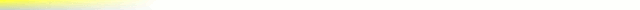# [mkgmap-dev] HGT - getElevation()

From Andrzej Popowski popej at poczta.onet.pl on Sun Jan 14 15:46:29 GMT 2018

```Hi Gerd,

> you just want to achive that the calculated postions are as close as
> possible to the positions in the hgt file

Yes. Linear interpolation is averaging too. If DEM can use exact values
form HGT, then there will be no averaging. Even interpolated values near
HGT node should be the same, because of rounding to 1m.

I have limited aligning to resolution better or equal 1200 and prepared
HGT area for worst case, which would be 1200. Patch attached.

--
Best regards,
Andrzej
-------------- next part --------------
Index: src/uk/me/parabola/imgfmt/app/dem/DEMFile.java
===================================================================
--- src/uk/me/parabola/imgfmt/app/dem/DEMFile.java	(revision 4056)
+++ src/uk/me/parabola/imgfmt/app/dem/DEMFile.java	(working copy)
@@ -22,6 +22,7 @@
import uk.me.parabola.imgfmt.app.ImgFileWriter;
import uk.me.parabola.imgfmt.fs.ImgChannel;
+import uk.me.parabola.imgfmt.Utils;

/**
* The DEM file. This consists of information about elevation. It is used for hill shading
@@ -52,9 +53,43 @@
* @param outsidePolygonHeight the height value that should be used for points outside of the bounding polygon
*/
public void calc(Area area, java.awt.geom.Area demPolygonMapUnits, String pathToHGT, List<Integer> pointDistances, short outsidePolygonHeight) {
-		HGTConverter hgtConverter = new HGTConverter(pathToHGT, area, demPolygonMapUnits);
+		double top = Utils.toDegrees(area.getMaxLat());
+		double bottom = Utils.toDegrees(area.getMinLat());
+		double left = Utils.toDegrees(area.getMinLong());
+		double right = Utils.toDegrees(area.getMaxLong());
+
+		// expand HGT area for aligning with resolution 1200
+		double hgtDis = 1.0D/1200;
+		double hgtTop = Math.ceil(top/hgtDis)*hgtDis;
+		double hgtBottom = Math.floor(bottom/hgtDis)*hgtDis;
+		double hgtLeft = Math.floor(left/hgtDis)*hgtDis;
+		double hgtRight = Math.ceil(right/hgtDis)*hgtDis;
+		Area hgtArea = new Area(hgtBottom, hgtLeft, hgtTop, hgtRight);
+
+		HGTConverter hgtConverter = new HGTConverter(pathToHGT, hgtArea, demPolygonMapUnits);
hgtConverter.setOutsidePolygonHeight(outsidePolygonHeight);

+		int res = hgtConverter.getHighestRes();
+		if (res >= 1200) {
+			if (pointDistances.get(0) == -1 || pointDistances.get(0) == (int) ((1 << 29) / (res * 45))) {
+				// align area with HGT raster
+				if (res == 1200) {
+					area = hgtArea;
+				}
+				else {
+					hgtDis = 1.0D/res;
+					top = Math.ceil(top/hgtDis)*hgtDis;
+					bottom = Math.floor(bottom/hgtDis)*hgtDis;
+					left = Math.floor(left/hgtDis)*hgtDis;
+					right = Math.ceil(right/hgtDis)*hgtDis;
+
+					area = new Area(bottom, left, top, right);
+				}
+				System.out.println("DEM aligned to HGT, resolution " + res);
+			}
+		}
+
int zoom = 0;
int lastDist = pointDistances.get(pointDistances.size()-1);
for (int pointDist : pointDistances) {
```